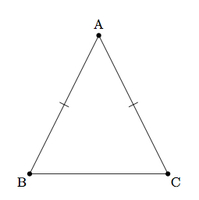# Congruent angles in isosceles triangles

Alignments to Content Standards: G-CO.C.10

Below is an isosceles triangle $ABC$ with $|AB| = |AC|$:Three students propose different arguments for why $m(\angle B) = m(\angle C)$.

1. Ravi says
If I draw the bisector of $\angle A$ then this is a line of symmetry for $\triangle ABC$ and so $m(\angle B) = m(\angle C)$.
2. Brittney says
If $M$ is the midpoint of $\overline{BC}$ then $\triangle ABM$ is congruent to $\triangle ACM$ and so $\angle B$ and $\angle C$ are congruent.
3. Courtney says
If $P$ is a point on $\overline{BC}$ such that $\overleftrightarrow{AP}$ is perpendicular to $\overline{BC}$ then $\triangle ABP$ is congruent to $\triangle ACP$ and so $\angle B$ and $\angle C$ are congruent.

Fill in the details in each argument to show why $m(\angle B) = m(\angle C)$. Can you find another different argument showing that $m(\angle B) = m(\angle C)$?

## IM Commentary

The goal of this task is to establish that base angles in an isosceles triangle are congruent. There are many approaches to the task, several of which are presented here. There are also many possible approaches to this task in the classroom:

• For a more directed approach, the teacher may wish to suggest one method or perhaps give different ideas to different groups of students.
• Students might be prompted without guidance to prove that base angles in an isosceles triangle are congruent. As they progress, the teacher can share some of the approaches given here.
• Students might discuss the relative merits of the different arguments. Which is easiest to follow? Which assumes the least background knowledge?

Two additional arguments are presented below, one very similar to Ravi's but using triangle congruence instead of reflections and the other similar to Courtney's argument but using trigonometric ratios instead of triangle congruence.

## Solution

1. Pictured below is the line $\ell$ which bisects angle $A$:Since $\ell$ contains $A$, reflection about $\ell$ maps $A$ to itself. Since $m(\angle DAB) = m(\angle DAC)$ we know that reflection about $\ell$ maps $\overrightarrow{AB}$ to $\overrightarrow{AC}$ and vice versa. Finally, reflections preserve distance as well as angles so this means that $B$ and $C$ are interchanged by this reflection. Since reflection over $\ell$ interchanges angles $B$ and $C$ we see that $m(\angle B) = m(\angle C)$.

2. The midpoint $M$ of $\overline{BC}$ is pictured below:Since $M$ is the midpoint of $\overline{BC}$ this means that $|BM| = |CM|$. We know by hypothesis that $|AB| = |AC|$. Since $|AM| = |AM|$ we can conclude by SSS that $\triangle ABM$ is congruent to $\triangle ACM$.  Since $\angle B$ and $\angle C$ are corresponding parts of congruent triangles they are congruent.

3. Suppose $P$ is the point on $\overline{BC}$ so that $\overleftrightarrow{AP}$ is perpendicular to $\overline{BC}$ as pictured below:By hypothesis $\angle BPA$ and $\angle CPA$ are right angles. We know that $|AC| = |BC|$ by hypothesis and $|AP| = |AP|$. By the Pythagorean theorem, $|BP| = |CP|$, and so by SSS $\triangle BPA$ is congruent to $\triangle CPA$. Hence $m(\angle B) = m(\angle C)$.

One alternate proof, building on the picture from Courtney's solution, uses trigonometric ratios. Using the picture above, we have $\sin{\angle B} = \frac{|AP|}{|AB|}$ and $\sin{\angle C} = \frac{|AP|}{|AC|}$. Since $|AB| = |AC|$ by hypothesis this means that $\sin{\angle B} = \sin{\angle C}$. Since these are both acute angles, this is only possible if $m(\angle B) = m(\angle C)$.

A second alternate proof builds on Ravi's picture. We have $|AC| = |AB|$ by hypothesis and $|AD| = |AD|$. We also know that $m(\angle BAD) = m(\angle CAD)$ by hypothesis. By SAS, $\triangle BAD$ is congruent to $\triangle CAD$ and we again conclude that $\angle B$ is congruent to $\angle C$.